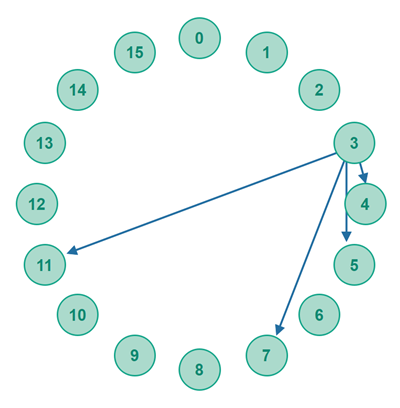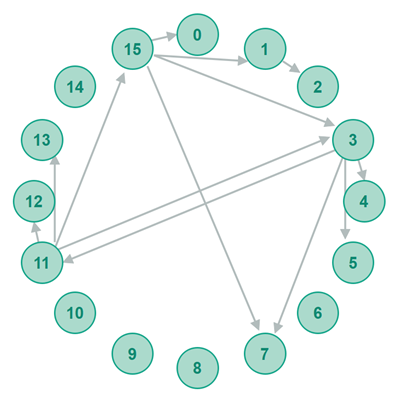# Chord P2P Network + DHT Algorithm

 Jakob Jenkov Last update: 2021-07-28

The Chord P2P network algorithm, and distributed hashtable (DHT) algorithm, is one of the earliest structured P2P network algorithms. Chord addresses peer addressability and peer findability and message routability challenges by organizing all peers in the P2P network into a single virtual ring. Each peer is assigned a unique GUID which is used for addressability, findability and message routability.

## Chord GUID Scheme

The GUID number space goes from 0 to [2 to the Nth power -1 => 2^N-1], where N is a number chosen by whoever starts the Chord P2P network. Using N = 8 would result in Chord GUIDs going from 0 to 255. Using N = 16 would result in Chord GUIDs going from 0 to 65535. You need to choose an N that is high enough that all peers expected to join the network can get a unique GUID. Common N values are 64, 128, 160 and up.

### Circular GUID Space

The Chord GUID space is considered to be circular, meaning 0 and 2^N-1 are considered to be neighbouring GUIDs. That means, that the distance between two GUIDs is directional. In other words, the distance as calculated from GUID A to GUID B is not the same as the distance calculated from GUID B to GUID A.

### Chord GUID Distance

In Chord, peers have a proximity which is calculated based on the distance between their GUIDs. Chord uses the numerical difference between the two GUIDs as distance. Here is a Chord GUID distance calculation example:

```GUID A = 2
GUID B = 15

distance(A, B) = B - A = 15 - 2 = 13
```

### Asymmetric GUID Distance

Because of the circular GUID space, calculating the distance between a GUID A and a GUID B varies depending on whether GUID A is larger than, or smaller than GUID B:

If GUID A is smaller than (or equal to) GUID B, then dist(A, B) = B - A

If GUID A is larger than GUID B, then dist(A, B) = B + 2^N - A

Here is calculation example:

```N = 4  = 16 GUIDs (0 to 15)

GUID A = 2
GUID B = 15

distance(A, B) = B - A       = 15 - 2      = 13

distance(B, A) = A + 2^N - B = 2 + 16 - 15 = 18 - 15 = 3
```

This asymmetric Chord peer GUID distance calculation example is also illustrated in the diagram below:## Chord Routing Table

For peers in a Chord network to be able to find each other, each peer needs to know about at least one other peer in the network. At the very least each peer needs to know the IP address (+ TCP or UDP port) of their nearest neighbour GUID-wise in the Chord network. This is illustrated in the diagram below:With only knowing the nearest neighbouring peer in the network it is possible to find any peer in the network. The lookup algorithm is very simple:

1. If the nearest neighbour is the peer (GUID) you are looking for - the lookup has finished successfully.

2. Else - ask the nearest neighbour to return either the address of the peer you are looking for, or the closest peer it knows to the target peer - if the neighbour doesn't know the target peer itself.

If the returned peer (GUID) is the peer you are looking for, the lookup has finished successfully.

If the neighbouring peer does not know any peer closer to the target peer than itself, it returns no peer info. The lookup has finished unsuccessfully.

Else, repeat 2) but send the request to the peer you just received from the previous lookup request.

Using this simple lookup algorithm, the peer searching will eventually ask all peers in the network, one at a time, until the peer it looks for is found, or the last peer says the closest peer it knows to the target peer is the searching peer itself - which will happen after a full round in the network.

Unfortunately, only knowing the nearest neighbour would result in a lookup time of O(N), meaning the lookup time will grow linearly with the number of peers in the Chord network. Chord has a solution for this problem.

### Referencing Exponentially More Distant GUIDs

To improve lookup time, the Chord routing table (also sometimes referred to as a finger table) is designed so that each peer keeps a pointer (entry) to more than just their nearest neighbour in the Chord network.

More specifically, each peer keeps references to peers that are exponentially more and more distant according to their GUID distance (from the referencing peer GUID). First, the routing table will contain a reference to the peer with a GUID distance of 1 from the referencing peer. Then a reference to the peer with a GUID distance of 2, then 4, then 8, then 16 etc. using the exponential distance of 2N with N going from 0 to the maximum N of the GUID space size (number of bits in a GUID). Here is an example:

A Peer Routing Table for Peer with GUID 3 (8 bit GUID size)
Entry IndexReferenced GUID  GUID Distance
041  (20)
152  (21)
274  (22)
3118  (23)
41916  (24)
53532  (25)
66764  (26)
7131128  (27)

The first 4 references are illustrated of the peer with GUID 3 is also illustrated in the diagram below. This diagram gives you a feeling of how the exponentially more distant references look in a virtual Chord ring (network):### Referencing Closest Peer to Target GUID

If no peer is found in the Chord network with a GUID that is exactly 2^N away in GUID distance, then the closest peer to that target reference GUID is stored instead.

For instance, if the target GUID to reference is 67, but there are only peers with GUID 66 and 69 in the network, then the peer closest to the target GUID will be referenced in that cell in the routing table instead.

In the above case it would be the peer with GUID 69, since dist(67, 69) = 2, whereas dist(67, 66) = 256 + 66 - 67 = 255 (because 66 is smaller than 67). Thus, 69 is closer to 67 than 66 is - because the distance from 67 to 69 and 66 has to be measured forward around the GUID "ring" (circle). This results in a distance of 2 to GUID 69, but a distance of 255 to GUID 66.

## Chord Peer Findability and Message Routability

When searching for a peer with a specific GUID (target GUID), the searching peer first looks in its own routing table for the target GUID. If the target GUID is found in its own routing table, the search is completed.

If the target GUID is not found in the searching peer's own routing table, the searching peer contacts the peer with the closest GUID to the target GUID - and asks that peer for the closest peer to the target GUID that it knows (has in its routing table). This process repeats until either the target GUID is found, or the searching peer detects that no peer exists in the network with the target GUID (if a peer responds that it does not know any peer with a GUID closer to the target GUID than itself).

The exponentially more distant GUID referencing scheme of the Chord routing table means two things:

1. Each peer knows more peers with GUIDs close to its own GUID than peers with more distant GUIDs.

2. Each peer knows another peer that is at most half the GUID space away (in GUID distance) from any target GUID in the whole Chord network.

The first characteristic means that the closer a searching peer gets to a given target GUID, the more peers the asked peer will know near the target GUID.

The second characteristic means that for every other peer a searching peer asks about a given target GUID, the remaining distance to the target GUID will be cut at least in half - every step of the search.

The second characteristic means that a searching peer is essentially performing a binary search of the Chord network when searching for a given GUID - by always at least cutting the remaining distance in half for every step in the search (for every peer asked about the peer with the closest GUID it knows to the target GUID). This means that a search in a Chord network for a specific GUID will have an execution time of O( log(N) ) .

Each step, each peer asked, is also sometimes referred to as a "hub", by the way.

### Chord Lookup Example

To better understand how the Chord lookup algorithm works (how Chord solves findability), let us look at a lookup example. In this example the peer with GUID 3 needs to find the peer with the GUID 2.

First, here is how the routing tables look for the peers involved in this specific lookup (peer 3, 11, 15, and 1):The lookup follows the following steps:

1. The peer with GUID 3 first looks inside its own routing table and find the peer with the closest GUID to 2. That is the peer with GUID 11.

2. Then the searching peer contacts the peer with GUID 11 and asks for the closest peer it knows to the peer with GUID 2. That is the peer with GUID 15.

3. The the searching peer contacts the peer with GUID 15 and asks for the closest peer it knows to the peer with GUID 2. That is the peer with GUID 1.

4. Then the searching peer contacts the peer with GUID 1 and asks for the closest peer it knows to the peer with GUID 2. That is the peer with GUID 2.

5. The lookup is now finished.

These steps are illustrated by the diagram below. The blue arrows mark the lookup steps.### Chord Routing Example

A Chord network could also route messages from a sending peer to a receiving peer along connections matching the entries in the peer routing tables.

Imagine that the peer with GUID 3 wants to send a message to the peer with GUID 2. Instead of looking up the IP + TCP / UDP port of the peer with GUID 2, as explained in the previous section, the peer with GUID 3 could have had the message routed via the Chord network. Routing the message from the peer with GUID 3 to the peer with GUID 2 would require the following steps:

1. The peer with GUID 3 forwards the message to the closest peer it knows to the peer with GUID 2. That is the peer with GUID 11.

2. The peer with GUID 11 forwards the message to the closest peer it knows to the peer with GUID 2. That is the peer with GUID 15.

3. The peer with GUID 15 forwards the message to the closest peer it knows to the peer with GUID 2. That is the peer with GUID 1.

4. The peer with GUID 1 forwards the message to the closest peer it knows to the peer with GUID 2. That is the peer with GUID 2.

5. The message routing is now finished.

Notice that the message would be forwarded along the same path as the lookup example in the previous section.

 Tweet Jakob Jenkov
Featured VideosClose TOCAll TrailsTrail TOCPage TOCPreviousNext